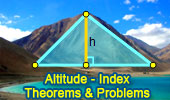# Altitude of a Triangle, Theorems and Problems - Table of Content 5Blanchet Theorem
Altitude.

Proposed Problem 29.
Right Triangle, altitude, incircle and inradius. Ten conclusions.

Proposed Problem 28
Right Triangle, altitude, incircles and inradius.

Proposed Problem 26
Right Triangle, altitude, incircles and inradius.

Proposed Problem 25
Right Triangle, altitude, incircles and inradius.

Proposed Problem 24
Right Triangle, altitude, incircles and inradii.

Proposed Problem 23
Right Triangle, altitude, incircles and inradii.

Proposed Problem 22
Right Triangle, altitude, incircles and inradii.

Proposed problem 21
Acute triangle, altitudes, orthocenter, diameter, tangents.

Proposed problem 20
Right Triangle, altitude, incircles and inradii.

Triangle and Squares 5
Altitude and median.

Triangle and Squares 3
Altitude.

Go to page: Previous | 1 | 2 | 3 | 4 | 5 | Next